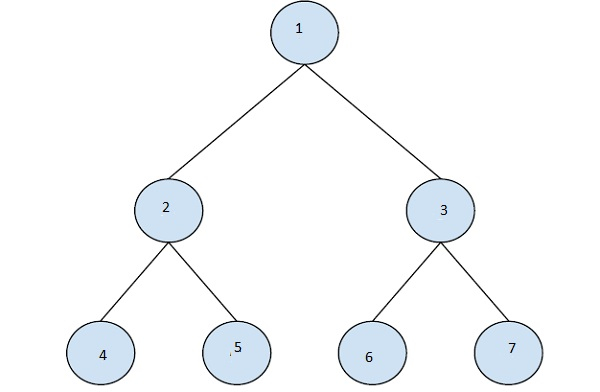# Find next right node of a given key in C++

C++Server Side ProgrammingProgramming

In this problem, we are given a binary Tree BT and a key value. Our task is to Find next right node of a given key.

Binary Tree is a special data structure used for data storage purposes.

Let’s take an example to understand the problem,

## Input

key = 4## Output

5

## Explanation

The element next to the node 4 is 5.

## Solution Approach

A simple solution to the problem is by traversing the binary tree using the level order traversal. And for the given key value, we will check if there exists an node next to node at the same level in the traversal. If Yes, return the next node, otherwise return NULL.

Program to illustrate the working of our solution,

## Example

Live Demo

#include <iostream>
#include <queue>
using namespace std;
struct node {
struct node *left, *right;
int key;
};
node* newNode(int key) {
node *temp = new node;
temp->key = key;
temp->left = temp->right = NULL;
return temp;
}
node* findNextRightNodeBT(node *root, int k) {
if (root == NULL)
return 0;
queue<node *> nodeVal;
queue<int> nodeLevel;
int level = 0;
nodeVal.push(root);
nodeLevel.push(level);
while (nodeVal.size()) {
node *node = nodeVal.front();
level = nodeLevel.front();
nodeVal.pop();
nodeLevel.pop();
if (node->key == k) {
if (nodeLevel.size() == 0 || nodeLevel.front() != level)
return NULL;
return nodeVal.front();
}
if (node->left != NULL) {
nodeVal.push(node->left);
nodeLevel.push(level+1);
}
if (node->right != NULL) {
nodeVal.push(node->right);
nodeLevel.push(level+1);
}
}
return NULL;
}
int main() {
node *root = newNode(1);
root->left = newNode(2);
root->right = newNode(3);
root->left->left = newNode(4);
root->left->right = newNode(5);
root->right->left = newNode(6);
int key = 4;
cout<<"The next right node of the node "<<key<<" is ";
node *nextNode = findNextRightNodeBT(root, key);
if(nextNode != NULL)
cout<<nextNode->key;
else
cout<<"not available";
return 0;
}

## Output

The next right node of the node 4 is 5